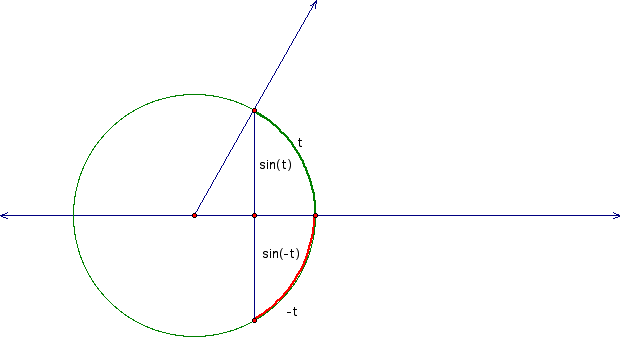## Trig Identities

An identity is an assertion about the equivalence of two expressions. To claim that two expressions are identical is to claim that the two expressions always have the same value.

Many trig identities are statements that can be seen as obvious just by looking at a diagram and understanding what the variables stand for. For example, knowing that t represents an arc length, the diagram below illustrates that sin(-t) = -sin(t).However, to see that this statement is always true you must imagine t varying through all its possible values and the statement always being true. For this it is helpful to have a dynamic visualization. Just click on the diagram above for a GSP sketch that does this.

Design modifications of this sketch (i.e., say how you would change it, don't actually change it) to support students seeing as many of the identities at this URL as you can. What conversation would you have with students about each one? What would you ask them to think about or try to see?